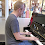## Friday, September 14, 2012

### Election Model: National Simulation

Simulating National Outcomes

National Elections are more about a national message than high variability in any given state. I simulate a national outcome first, then simulate individual state outcomes based on both the national outcome and how each individual state tends to perform relative to the national outcome (for example, Vermont currently looks 17 points better for Obama than the national outcome, Alabama looks 21 points worse). Simulating in this manner ensures that states tends to move together with the national outcome, but are allowed to vary individually.

Each individual election is simulated by drawing a random sample from a normal distribution, then the results are aggregated to give summary statistics (for example, Obama won the Electoral Vote 84.1% of the time the last time I ran 25,000 simulations). The mean and variance of the distributions are calculated as follows:

The mean is based on polling average. For national outcomes the mean is simply the current polling average. For state outcomes it is the national poll average + the state adjustment (+17 for Vermont, -21 for Alabama).

Variance is more difficult and I've struggled with it. I ultimately decided on the following. Using the calibrated logistic function I believe I've arrived a way of translate polling advantage, sample size, and distance to the election into a reliable winning percentage in a way that is cognizant of the 3 primary sources of error. In calculating the appropriate variance to use I leverage that calculation. Given that I know an average polling outcome (say, Obama +8.9 for Connecticut) and the probability Obama wins that state (if the state election were an independent event) in this case 98.67%. Using those two numbers I can calculate an implied polling standard deviation (4.02% in the case of CT

The detailed outcomes from my 9/13 simulation are below.

1.1.